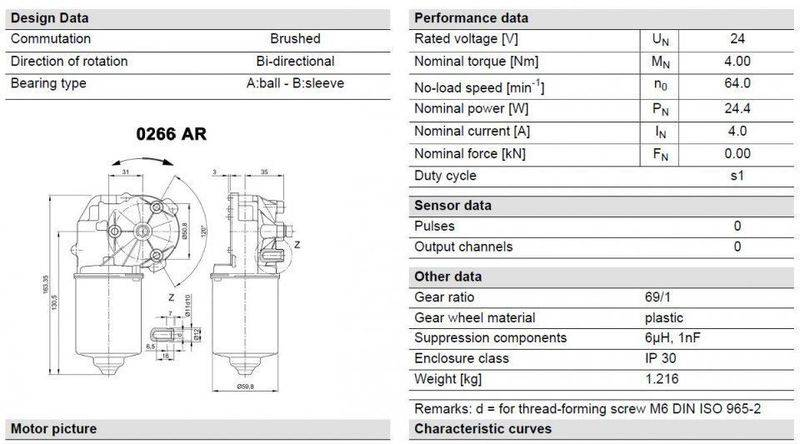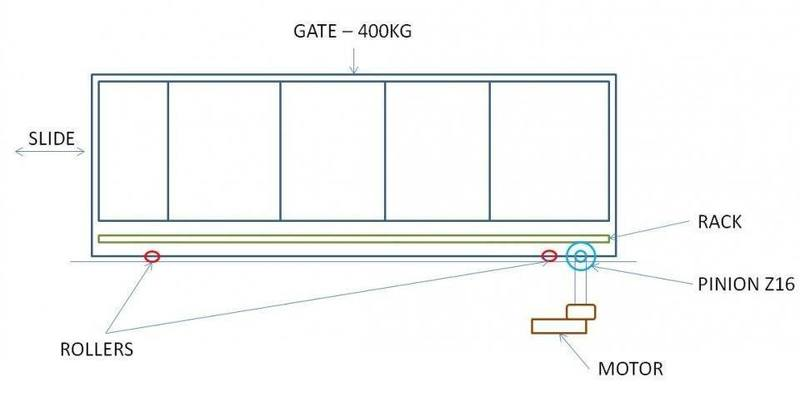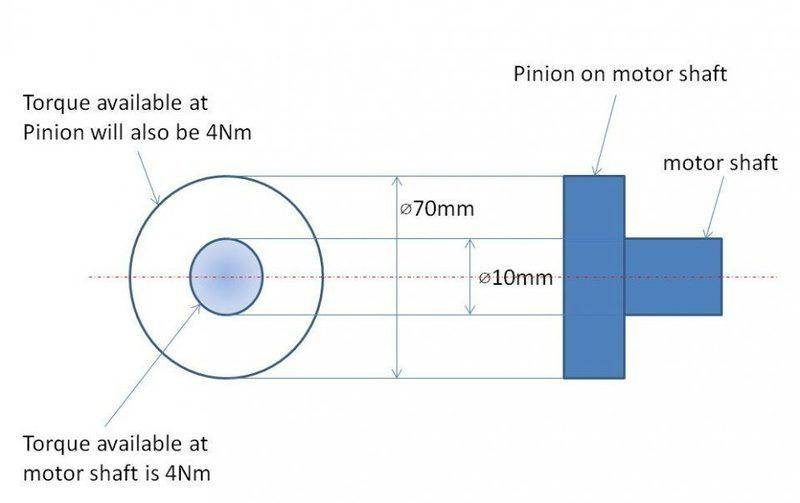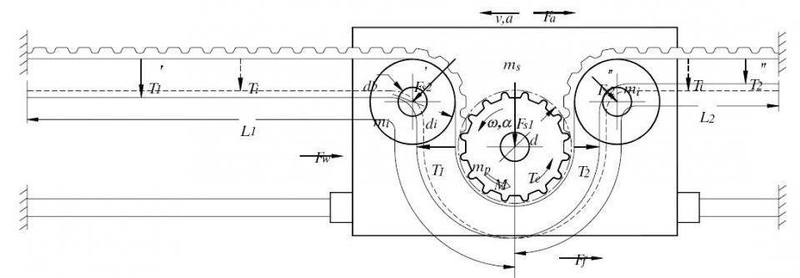# Torque & Force required to slide a gate

Greetings all,

I am trying to build a sliding gate operator. I have this wiper motor which says, 24v dc, 64rpm, nominal torque- 4Nm.Max weight of gate to be 400kg, sliding on a steel rail (Not sure how to consider rolling resistance or Co-eff of friction) . Max pull force to be achieved by motor is 550N. Rack & Pinion is used to transmit force from motor to gate. velocity to be 9 cm/sec worst case.

Pinion = Z16

All i need to do is validate that the above motor is capable of pulling a 400kg gate.## Answers and Replies

Simon Bridge
Science Advisor
Homework Helper
Welcome to PF;
Due to the possible liability issues, and the possible impact of regulations in your jurisdiction - you should ask an engineer.
You will probably be able to find one who specializes in motorized gates.

Note: the force to move the gate depends on what is opposing the motion and how fast you want the gate to accelerate.
To keep the gate moving at a constant speed takes only the force to overcome resistance.

I need proper equations and I need to understand it.

All i have is a torque eqn. T=F*r

So, 4Nm (o/p torque) = F * 0.005m (radius of o/p shaft)

i get F=800N at the motor o/p shaft (after gear reduction)

And i have another question.

- If 4Nm torque is available at 0.005m shaft, what if I mount a pinion of radius 0.035mm, will that have the same 4Nm torque or will it be reduced

Simon Bridge
Science Advisor
Homework Helper
The torque is the same... youll get more fotce at the end of a longer arm, but the motor will have a limited power.

Power is work per unit time, work is torque times angle rotated... so power at constat speed would be torque times angular velocity.

The torque is the same... youll get more fotce at the end of a longer arm, but the motor will have a limited power.

Power is work per unit time, work is torque times angle rotated... so power at constat speed would be torque times angular velocity.

So what I understand is that same torque will be available at pinion i.e. 4Nm.But there will a reduction in force.

Calculation from 3rd post says output force will be 800N

So when calculating for pinion i.e. T=F*r
4Nm= F * 0.035m
F = 114.28N (Force too less than the force required to pull the gate)

But required force is 550N

Am i going wrong some where ?.

Thank you

jack action
Science Advisor
Gold Member
The conversion from torque to force is F = T * (GR / r) , where GR is the gear ratio of a gearbox (belt & pulleys, chain & sprockets, or whatever) and r is the radius of your pinion. The speed of your rack will be v = ω * (r / GR), where ω is the angular speed of your motor.

Assuming the motor produces 4 N.m @ 64 rpm (6.7 rad/s) and you want F = 550 N, then (GR / r) = 550 / 4 = 137.5 m-1. Then your rack speed will be v = 6.7 / 137.5 = 0.049 m/s or 4.9 cm/s. If this is not fast enough for you, you need more power. Because, all you really need to know is how much power the motor can deliver. How much power do you need? P = F * v. Since you already know F, and you said worst case scenario is 9 cm/s, then the motor needs at least 550 * 0.09 = 49.5 W to do the required work.
All i need to do is validate that the above motor is capable of pulling a 400kg gate.
Assuming the motor cannot give more than 24.4 W of power, no, not with the requirement you specified (force and speed).

•James Nilsen-Misra and Merlin3189
The conversion from torque to force is F = T * (GR / r) , where GR is the gear ratio of a gearbox (belt & pulleys, chain & sprockets, or whatever) and r is the radius of your pinion. The speed of your rack will be v = ω * (r / GR), where ω is the angular speed of your motor.

Assuming the motor produces 4 N.m @ 64 rpm (6.7 rad/s) and you want F = 550 N, then (GR / r) = 550 / 4 = 137.5 m-1. Then your rack speed will be v = 6.7 / 137.5 = 0.049 m/s or 4.9 cm/s. If this is not fast enough for you, you need more power. Because, all you really need to know is how much power the motor can deliver. How much power do you need? P = F * v. Since you already know F, and you said worst case scenario is 9 cm/s, then the motor needs at least 550 * 0.09 = 49.5 W to do the required work.

Assuming the motor cannot give more than 24.4 W of power, no, not with the requirement you specified (force and speed).

Thanks JACK. So according to your calculation the fore of 550N can be achieved with a 4Nm torque but there would be a compromise on velocity i.e. 4.9 cm/s.

Since I am using a Rack & Pinion to transmit power what would be the gear ratio?. I came across many calculations and posts where there is no Gear ratio for Rack and Pinion. (Keeping in mind lenth of the Rack will vary according to gate length)

Thank you.

jack action
Science Advisor
Gold Member
So according to your calculation the fore of 550N can be achieved with a 4Nm torque but there would be a compromise on velocity i.e. 4.9 cm/s.

That is the whole concept of power. Power can be switched back and forth between force and velocity (or torque and rpm) at will. If you want to achieve a certain force at a certain speed, you need a certain amount of power.

Since I am using a Rack & Pinion to transmit power what would be the gear ratio?. I came across many calculations and posts where there is no Gear ratio for Rack and Pinion. (Keeping in mind lenth of the Rack will vary according to gate length)

A rack & pinion doesn't have a gear ratio per say. I included one because I noticed that it might be physically impossible to construct a system that respected your criteria without the addition of a gearbox between the motor and the rack & pinion.

In my calculation, I got 137.5 m-1 for th (GR / r). r would be the radius of the pinion. If we assume GR = 1 (the motor directly connected to the rack & pinion), then the radius of the pinion needs to be 1/137.5 m or a diameter of 14.5 mm. I don't think you will find a rack & pinion with such a small gear that will support 550 N (but I haven't checked anything). But if you put a gearbox between the two and, say, it has a GR of 10, then the radius needed becomes 10/137.5 m or a diameter of 145 mm. Whether you use one set up or the other (or any valid combination of GR / r), you will always obtain a force of 550 N at 4.9 cm/s.

Thanks a lot Jack

If u see the motor data sheet, It is already coupled with a gear box. The torque of the motor alone is 0.05Nm (According to my calc) but at the output shaft we get 4Nm after torque step up through gear box ( worm and worm wheel arrangement ). I think it would be better to go for a motor with higher torque.

But the current motor was capable of pulling 550N at 9 cm/s through a "toothed pulley and Belt" (similar to the below setup). Does this make any difference with rack and pinion?The nominal power rating is the continuous output mechanical power rating. Typically that will be the rating for about 25% efficiency for small electric motors.

From the motor data sheet, the input electrical current and voltage ratings are 4 A and 24 V respectively. The nominal input electrical power then is 96 Watts and at 24.4 Watts mechanical output that is right about the 25% efficiency so that makes sense.

For short duty cycles, such as opening and closing a gate, you can probably run the motor at higher efficiency, up to around 50% or so.

So if your calculation of .09 m/s at 550 N is correct, that is 49.5 Watts and your motor may be sufficient for this application but you may want to consider using one with higher ratings.

•James Nilsen-Misra and asoundha
jack action
Science Advisor
Gold Member
But the current motor was capable of pulling 550N at 9 cm/s through a "toothed pulley and Belt" (similar to the below setup). Does this make any difference with rack and pinion?
No, same thing.

•asoundha
Sorry to be negative, but I don't think so.

I can give you three types of reasons why this motor very probably won't work:-

1. Experience. I've actually used very similar motors for some stuff. Hold it in your hand, look at it and then try to envisage a half ton steel gate. It's half the weight of a small car (admittedly a British car). If you have engineering experience, you'll feel (whoo-oo) that the motor is way under sized. 40kg, yes. 400kg, no.

2. Engineering. The torque calculations answer a very hypothetical and mathematical force translation in an ideal scenario. In the field,
a. The force to roll the gate is irrelevant. You need to overcome the static friction to start it moving. The primary resistance will come from the rollers. I don't know what you plan to use but imagine them operating in two years time, covered in crud, caked grease , dust and rust. Also consider the condition of the running surface and what might be covering it. If they're not metal rollers, additional rolling resistance will be generated. Also, what's holding the gate upright? Will you have lateral rollers or rely on friction with gate posts? Latter comments apply to both.
b. The pinion will not apply force horizontally to the gate. It will be at an angle dependant on the teeth's pressure angle and how well you can locate the pinion's pitch line with respect to that of the rack's. The pitch circle diameter of the pinion will be smaller than 70mm, maybe down to 55mmish. If you operate the pinion as drawn (below the rack), you risk the gate bouncing over the pinion teeth much as a screw driver does bouncing around a cross point screw. In the alternative arrangement (pinion on top) you'll need significant downward restraint to prevent the motor doing the same.
c. The torque on the motor shaft is compounded by the asymmetrical nature of the drive. There will be a considerable bending moment on the shaft equivalent to the force multiplied by the distance between the centroids of the pinion and the gearbox bush (plastic). It would be interesting to know if your belt drive arrangement incorporated a radial bearing on the other side of the belt. You could incorporate one into the motor housing, but then that would require a structural design for the applied loading onto the housing. You should really have a radial bearing the motor side of the pinion as well. Bushes are not designed for shaft bending.

3. The market. I had a look at a British gate closer supplier. They sell 600 kg gate kits with 350W motors weighing 7kg.

As a piece of advice that's not worth the electricity it took to write, I suggest the following with risk mitigation in mind:-

1. Research the motor market for specifications of 350W motors.
2. Design your gate and closure mechanism for a motor rated to 350W.
3. Construct and install the gate.
4. Close gate - put a set of bathroom scales against the gate post - jack the gate open with car jack braced off the scales to estimate the actual force.
5. Add 50 - 100% extra power.
6.. Install the motor and commission.

Remember, the costs of the additional power will be eclipsed by the hassle /embarrassment /scolding from wife when you have to upgrade the motor and mounting.

Last edited:
•jack action and billy_joule
The nominal power rating is the continuous output mechanical power rating. Typically that will be the rating for about 25% efficiency for small electric motors.

From the motor data sheet, the input electrical current and voltage ratings are 4 A and 24 V respectively. The nominal input electrical power then is 96 Watts and at 24.4 Watts mechanical output that is right about the 25% efficiency so that makes sense.

For short duty cycles, such as opening and closing a gate, you can probably run the motor at higher efficiency, up to around 50% or so.

So if your calculation of .09 m/s at 550 N is correct, that is 49.5 Watts and your motor may be sufficient for this application but you may want to consider using one with higher ratings.

Thanks a lot Tom,

By the way the above data sheet has incorrect information about the motor. I had a chance to look at the motor carefully where in I noticed the sticker, which says 6 Amps, 24v, 34RPM, 36W. I do not know why, but I am considering the spec. on the motor.

Seriously I never thought about Motor Duty cycle. You where so correct, these little motors for my purpose can be run at up-to 50% Duty cycle. I calculated the output torque at 50% Duty cycle and it is around 20 Nm which is really good. I need to work on it and get this completed by using the same motor.

Cheers
Thanks again.... :) :)